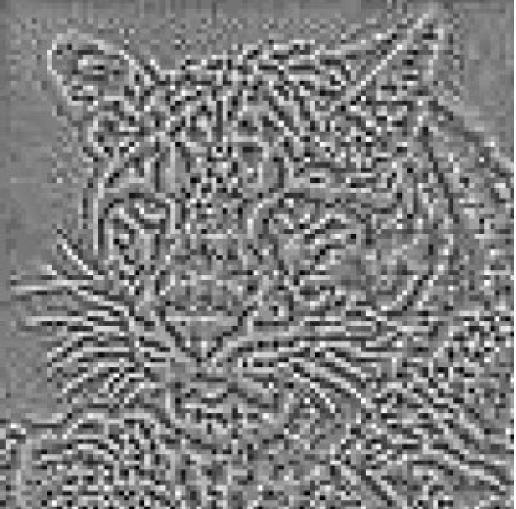# Numeric Solutions of Eigenvalue Problems for Generic Nonlinear Operators

### Ester Hait-Fraenkel, Guy Gilboa, arXiv

Numerical methods for solving linear eigenvalue problem are widely studied
and used in science and engineering. In this paper, we propose a generalized
numerical method for solving eigenproblems for generic, nonlinear opera-
tors. This has potentially wide implications, since most image processing
algorithms (e.g. denoising) can be viewed as nonlinear operators, whose
eigenproblem analysis provides information on the most- and least-suitable
functions as input. We solve the problem by a nonlinear adaptation of the
power method, a well known linear eigensolver. An analysis and valida-
tion framework is proposed, as well as preliminary theory. We validate the
method using total-variation (TV) and demonstrate it on the EPLL denoiser
(Zoran-Weiss). Finally, we suggest an encryption-decryption application.
Keywords: eigenfunctions, nonlinear operators, denoising, power iteration,
total-variation, EPLL.